IF YOU CAN SEE THIS go to /sfa/Nuprl/Shared/Xindentation_hack_doc.html

### Propositions

The topic of how propositions are treated in type theory has many aspects, some rather subtle. We start with superficial aspects, which may suffice for most users. Here are some of the usual predicates and connectives.

 P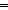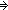Q [if P then Q] P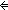Q [P if and only if Q] P & Q [P and Q] P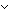Q [P or Q]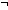P [not P] False [a canonical false proposition] True [a canonical true proposition]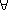x:A. P(x) [for every x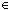A, P(x)]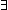x:A. P(x) [there is some xA such that P(x)] a = bA [a is the same member of A as b; the type may be elided] a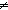bA ab a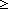b [relations between integers]

Rather than interpret propositional formulas as having Boolean values, i.e. as being either true or false, we use a type "Prop" of "propositional values". (This is because users of Nuprl usually want their results to be constructively or intuitionistically meaningful, which precludes the classical Boolean-valued interpretation of propositions.) For example,

Thm*i,j: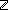. (i<jProp
Thm*T:Type, a,b:T. (a = bTProp
Thm* FalseProp
Thm*A:Prop. (AProp
Thm*A,B:Prop. (ABProp
Thm*A,B:Prop. (A & BProp
Thm*A,B:Prop. (ABProp
Thm*A:Type, P:(A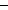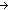Prop). (x:AP(x))Prop
Thm*A:Type, P:(AProp). (x:AP(x))Prop

Observe that we treat properties, relations, connectives and quantifiers as Prop-valued Functions. Propositional connectives take Prop's as arguments. Quantifiers such as "x:AP(x)" and "x:AP(x)" take two arguments, one being the "domain of quantification", and the other being a property (i.e. Prop-valued function) defined on that domain. As indicated by the forms of these theorems, one can quantify over (some) propositions and properties, thus, the Nuprl language is higher-order. See Higher-Order Propositions.

Two type constructors having propositional components are {x:AB(x) } and T(given P), which are discussed in Comprehension Subtypes and Guarded Types. There is, however, no "place" in computational type theory for a "choice" operator such as David Hilbert's, which takes any type having a member and denotes a member of it, because, as explained in Types, the method for computing any such purported choice operations would have to have been already defined independently of the types.

The heart of intuitionistic type theory, such as Martin-Lof's or Nuprl's, is to account for some "computational content" of propositions, expressed as types of such content. Thus, there is a sense in which one can represent Propositions as Types.

Finally, there is another distinct notion of proposition, namely the content of what one may assert in Nuprl Proofs. Those forms of assertion used are codified as Sequents, and are designed to help us reason about types and about propositions in the above sense. In type theory one normally reserves the nomenclature "Proposition" for the notion employed above, and adopts some other term such as "possible judgement" or "form of judgement" for the other; in Nuprl we tend simply to use "sequent" since it is the form we have adopted for such forms of assertion in Proofs.

(Sept 2003 - sfa )